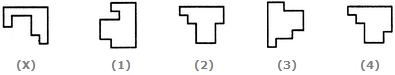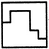# Non Verbal Reasoning - Shape Construction - Discussion

### Discussion :: Shape Construction - Section 3 (Q.No.8)

In each of the following questions, a key figure marked (X) is given, followed by four other alternative figures marked (1), (2), (3) and (4). It is required to select one figure from the alternatives, which fits exactly into figure (X) to form a complete square.

8.

Select a figure from the given four alternatives which fits exactly into Figure-X to form a complete square.[A]. 1 [B]. 2 [C]. 3 [D]. 4

Explanation:Indhuja said: (Mar 6, 2017) I can't understand this. Can anyone please explain this?